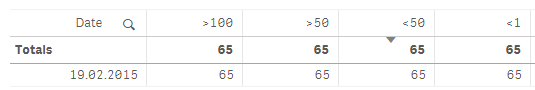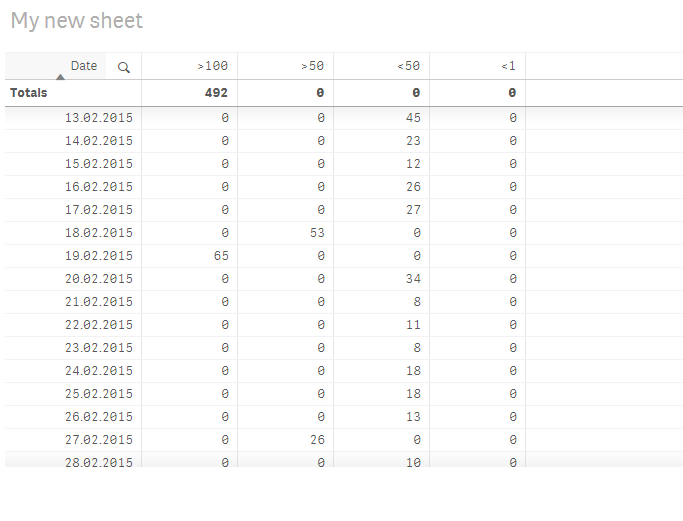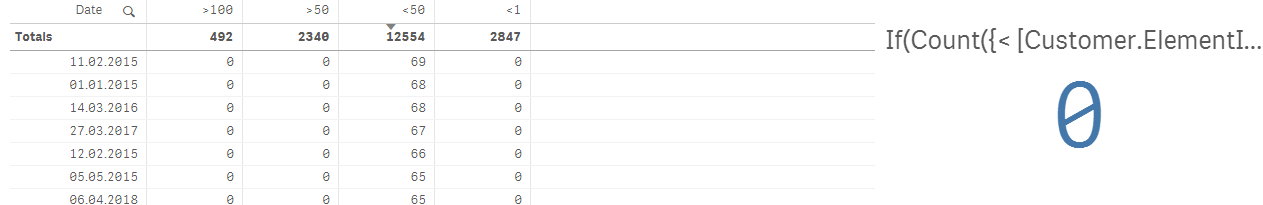# New to Qlik Sense

If you’re new to Qlik Sense, start with this Discussion Board and get up-to-speed quickly.

Announcements
cancel
Showing results for
Did you mean:Creator

## IF greater, else zero

Qlik experts,

Hopefully someone can help me with an IF-statement or set-analysis.

I want to display calculations dependent on a record if >100, than count ID. The same for the following criteria - >50, <50 and <1.

My problem is that, if one record has >100, there is also caunt for ID for >50, <50 and <1. These should be set to zero. If there is a record with greater than 100, the rest should be zero.

Count({< [Customer.ElementID]= {'RR'}, [Customer.Value]={">100"}>} distinct [Product.Prod_ID])1 Solution

Accepted SolutionsMVP

Something like this?8 RepliesMVP

Something like this?Creator
Author

Thanks sunny!

It’s almost perfect. I see that my calculations would not work for <1 due to the <50 will also include 0.4 values. Is there an expression for less than 50 and greater than 1 (<50 and >1)?MVP

I think you may want to change < 50 to >=1Creator
Author

Perfect!Creator
Author

Sunny,

I have one more question that hopefully you can help me with. Can these expressions not be used in KPI? I want the KPI to display the sum, like the table does at the top line.MVP

For these to work in KPI, wrap the expression around with Sum(Aggr(...)) function

Sum(Aggr(YourExpression, Date))Creator
Author

sum(Aggr(Count({< [Customer.ElementID]= {'RR'}, [Customer.Value]={">100"}>} distinct [Product.Prod_ID]) +

Count({< [Customer.ElementID]= {'RR'}, [Customer.Value]={">50"}>} distinct [Product.Prod_ID]) = 0,

Count({< [Customer.ElementID]= {'RR'}, [Customer.Value]={"<50"}>} distinct [Product.Prod_ID], Date), 0))

This is the expression used in the table:

If(Count({< [Customer.ElementID]= {'RR'}, [Customer.Value]={">100"}>} distinct [Product.Prod_ID]) +

Count({< [Customer.ElementID]= {'RR'}, [Customer.Value]={">50"}>} distinct [Product.Prod_ID]) = 0,

Count({< [Customer.ElementID]= {'RR'}, [Customer.Value]={"<50"}>} distinct [Product.Prod_ID]), 0)MVP

For > 50... you will use this

Sum(Aggr(

If(Count({< [Customer.ElementID]= {'RR'}, [Customer.Value]={">100"}>} distinct [Product.Prod_ID]) = 0,

Count({< [Customer.ElementID]= {'RR'}, [Customer.Value]={">50"}>} distinct [Product.Prod_ID]), 0)

, Date))

For >= 1... you will use this

Sum(Aggr(

If(Count({< [Customer.ElementID]= {'RR'}, [Customer.Value]={">100"}>} distinct [Product.Prod_ID]) +

Count({< [Customer.ElementID]= {'RR'}, [Customer.Value]={">50"}>} distinct [Product.Prod_ID]) = 0,

Count({< [Customer.ElementID]= {'RR'}, [Customer.Value]={">=1"}>} distinct [Product.Prod_ID]), 0)

, Date))

and for < 1... you will use this

Sum(Aggr(

If(Count({< [Customer.ElementID]= {'RR'}, [Customer.Value]={">100"}>} distinct [Product.Prod_ID]) +

Count({< [Customer.ElementID]= {'RR'}, [Customer.Value]={">50"}>} distinct [Product.Prod_ID]) +

Count({< [Customer.ElementID]= {'RR'}, [Customer.Value]={">=1"}>} distinct [Product.Prod_ID]) = 0,

Count({< [Customer.ElementID]= {'RR'}, [Customer.Value]={"<1"}>} distinct [Product.Prod_ID]), 0)

, Date))